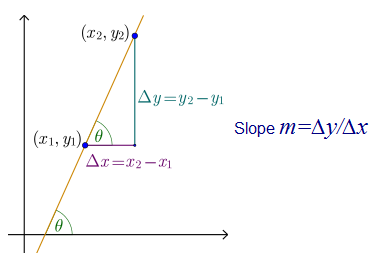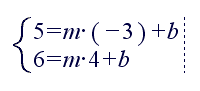# Blog

Linear Equation and Slope of a Line using UMS

# 部落格

Linear Equation and Slope of a Line using UMS

# Blog

Linear Equation and Slope of a Line using UMS

# Блог

Linear Equation and Slope of a Line using UMS

# Blog

Linear Equation and Slope of a Line using UMS

# Blog

Linear Equation and Slope of a Line using UMS

# בלוג

Linear Equation and Slope of a Line using UMS

# Blogue

Linear Equation and Slope of a Line using UMS

# Blog

Linear Equation and Slope of a Line using UMS

# Blog

Linear Equation and Slope of a Line using UMS

## Linear Equation and Slope of a Line using UMS

linear equation is an algebraic equation in which each term is either a constant or the product of a constant and (the first power of) a single variable. Linear equations can have one or more variables.

One variable
A linear equation in one unknown x may always be rewritten ax=b If  a≠0, there is a unique solution x=b/a If  a≠0 , then either the equation does not have any solution, if b≠0 (it is inconsistent), or every number is a solution, if b is also zero.

Two variables
A common form of a linear equation in the two variables x and y is y=mx+b, where and b designate constants (parameters). The origin of the name “linear” comes from the fact that the set of solutions of such an equation forms a straight line in the plane. In this particular equation, the constant m determines the slope or gradient of that line, and the constant term b determines the point at which the line crosses the y-axis, otherwise known as the y-intercept.

General (or standard) form
In the general (or or standard ) form the linear equation is written as: Ax+By+C=0 where A and B are not both equal to zero. The equation is usually written so that A>0 , by convention. The graph of the equation is a straight line, and every straight line can be represented by an equation in the above form. If A is nonzero, then the x-intercept, that is, the x-coordinate of the point where the graph crosses the x-axis (where, y is zero), is C/A. If B  is nonzero, then the y-intercept, that is the  y-coordinate of the point where the graph crosses the y-axis (where x is zero), is C/B, and the slope of the line is -A/B. The general form is sometimes written as: ax+by+c=0 where a and b are not both equal to zero. The two versions can be converted from one to the other by moving the constant term to the other side of the equal sign.

Intercept form
x/a+y/b=1, where a and b must be nonzero. The graph of the equation has x-intercept a and y-intercept  b. The intercept form is in standard form : Ax+By+C=0 with  A/B=1/a and  B/C=1/b  . Lines that pass through the origin or which are horizontal or vertical violate the nonzero condition on a  or b and cannot be represented in this form.

Find The Slope of a Line
In mathematics, the slope or gradient of a line is a number that describes both the direction and the steepness of the line . Slope is often denoted by the letter m. If the equation of the line is y=mx+b, then m is the slope.Questions

1) How to find the slope of a line given 2 points (-3;5) , (4;6) using UMS ?

The equation of the line is y=mx+b, m is the slope
First point of the line is (-3;5), or x=-3, y=5
Second point of the line is (4;6) or x=4, y=6
EnterSolve using UMS and find m

2) How to find the slope of a Line : 6x+2y=10 using UMS ?

The equation of the line is y=mx+b, m is the slope
Enter : 6x+2y=10 and solve using UMS for y.
We get y=-3x+5

3) How to find the slope of a Line x/5+3/y=4 using UMS ?

The equation of the line is y=mx+bm is the slope.
Enter x/5+3/y=4 and solve using UMS for y
We get y=-4(x-5)/15 therefore y=-4/15x+20/15

4) How to find the slope of a Line : 3x-7y+5=0 using UMS ?

The equation of the line is y=mx+bm is the slope.
Enter : 3x-7y+5=0 and solve using UMS for y
We get y=(3x+5)/7 therefore y=3/7x+5/7

### 标签

For Windows OS

pour Windows

для Windows OS

voor Windows

für Windows Betriebssysteme

per Windows OS

para Windows OS

למערכת הפעלה ווינדוס

Windows İşletim Sistemi için

### פתור ותרגל שיעורי בית במתמטיקה עכשיו!

למערכת הפעלה ווינדוס

для Windows OS

### Matematik ödevinizi hemen şimdi çözün!

(Windows İşletim Sistemi için)

Pour Windows

Per Windows OS

Para Windows OS

### Löse und übe jetzt deine Mathe-Hausaufgaben!

Für Windows Betriebssysteme

Voor Windows

For Windows OS